Courses

# Equations of State for Real Fluids Civil Engineering (CE) Notes | EduRev

## Civil Engineering (CE) : Equations of State for Real Fluids Civil Engineering (CE) Notes | EduRev

The document Equations of State for Real Fluids Civil Engineering (CE) Notes | EduRev is a part of the Civil Engineering (CE) Course Thermodynamics.
All you need of Civil Engineering (CE) at this link: Civil Engineering (CE)

Equations of State for Real Fluids

The generic form of an equation of state (EOS) is: f ( P,V , T ) = 0

However, as we have already seen by the phase rule, for a single phase pure component the degrees of freedom are two. This may be expressed in the form of an EOS equation as follows:

V = V (T, P)

It follows that: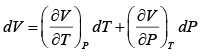... (2.2)

Defining Volume Expansivity as β =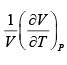...(2.3)

Isothermal compressibility as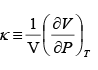...(2.4)

The generic EOS (2.2) may be written as: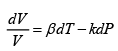2.3.1 EOS for Liquids

For liquids, which are relatively incompressible, the factors β and κ are generaly show an weak dependence on T and P and hence averaged values of these parameters may be used for

estimating the liquid volume at any temperature using the following integrated form of the equation (2.5):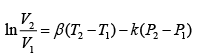For liquids the usual datum volume (i.e., V1 in eqn.2.6) can be the saturated volume at a given temperature, which may be obtained from the Rackett equation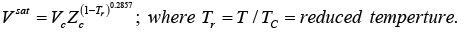Where ZC is the critical compressibility factor

EOS for Gases

In contrast to liquids, gases are relatively far more compressible, and so volume is strongly dependant on temperature and pressure. Consequently eqn. 2.6 cannot be used easily to estimate volume at a given T & P, as both β and κ are strong functions of T and P. Thus, various EOSs have been proposed to describe gas phase volumetric properties. The next section presents select EOSs that are typically used for the gas phase, ranging from those applicable to moderate pressure to others which are more accurate at high pressures.

Virial EOS:

Generally applicable to moderate deviations from ideal gas behavior, the virial EOS is given by two alternate forms: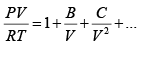...(2.8)

Where B, C = second and third virial coefficients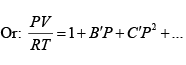...(2.9)

It may be shown that the following relations hold further: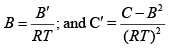...(2.10)

As may be evident, the second and third terms on the right side of eqns. 2.8 and 2.9 constitute corrections for the non-ideal behavior of a gas. The virial coefficients are essentially dependent of temperature. The more the number of virial coefficients used in the equation the better is the prediction of gas molar volume. While the estimation of the second virial coefficient is relatively straightforward, that of the third virial coefficient is generally far more complex, and there is scant data in the literature on its value for different substances. Because of this the virial EOS is most commonly used in the truncated form, and applies to moderate deviations from ideal gas behavior: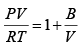...(2.11)

Alternately,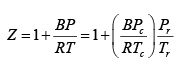...(2.12)

Where, Pr and Tr are reduced pressure and temperature respectively, given by: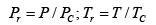A set of generalized correlations have been proposed by Pitzer and co-workers for computing the second virial coefficients where by

l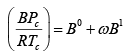...(2.13)

The terms B 0 and Bare given by: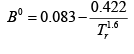...(2.14)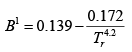...(2.15)

The parameterω , discussed in a later section, is the acentric factor, which is a fundamental thermodynamic property of a substance, and is a measure of asphericity of molecular shape . The value of acentric factor may be obtained from the following expression from experimental vapour pressure data of a pure substance

ω ≡−1.0 − log ( Prsat )Tr =  0.7                           ...(2.16)

( Prsat )Tr =  0.7 = reduced saturation vapour pressure at a reduced temperature equalling 0.7.

Since the virial EOS applies to only moderate deviations from ideal behaviour several complex extensions of it have been proposed for increasing accuracy of prediction at higher pressures; an example of such an EOS is the so-called Benedict-Webb-Rubin (BWR) EOS (G.B.Benedict, G. B. Webb, and L. C. Rubin, J. Chem. Phys., vol. 8, pp. 334-345, 1940) used in the petroleum and natural-gas industries: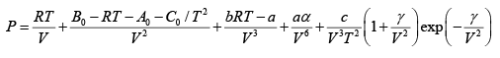...(2.17)

where A 0 , B , C , a, b, c, andγ are all constant for a given fluid.

Cubic EOS for Gases:

While the truncated virial EOS applies to moderate pressures, it is often necessary to obtain volumetric properties of gases at relatively much higher pressures. It has generally proved to be computationally unwieldy to use extensions of the virial EOS at higher pressure. To obviate this problem an entire range of alternate EOSs, termed cubic EOS, have been proposed by various workers. The term “cubic equation of state” implies an equation which, if expanded, would contain the volume terms raised to the first, second, or third power. Such an EOS provides two distinct advantages over many other more complex EOS found in the literature; they allow:

• Prediction of both gas and liquid (saturated) phase molar volumes.
• Provide a trade-off between complexity and accuracy of prediction

The first of such cubic EOS was proposed by the Dutch physicist Johannes Diderik van der Waals in 1873, and has the following form: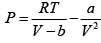...(2.18)

Where, a and b are characteristic constants for a pure substance. For example, for nitrogen the values of a and b are 0.1368Pa.m6 / mol2 and 3.864x10−5m3 / mol respectively.
Once can take advantage of the phase P-V behaviour at the critical point (see fig. 2.1) to determine the expressions for a and b. Since the P-V curve offers a point of inflection at the critical point one may write: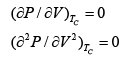On applying eqns. (2.19) and (2.20) on 2.18 two independent equations obtain, which may solved simultaneously to show that for the vdW EOS:

a = (27 / 64) R2 2T2C / PC

b = (1 / 8) RTC / PC

VC =(3 / 8) / RTC/ P

It follows that the critical compressibility factor is then given by: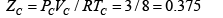The vdW EOS suggests that for all pure substances the critical compressibility factor is a constant (= 0.375). This, however, is contrary to experience. Indeed, although similar in magnitude, the value of ZC is specific to a pure substance. For example, for methane it is 0.286, while for helium 0.302. This indicates that although an improvement over ideal gas EOS, the vdW EOS does not reflect the specificity needed to predict the behaviour of all substances at high pressures.

To bridge this gap several other cubic EOS of higher accuracy have been advanced, the three most widely used of which are:

• Redlich-Kwong (RK) EOS (Otto Redlich and J. N. S. Kwong, Chem. Rev.,vol. 44, pp. 233-244, 1949)
• Soave-Redlich-Kwong (SRK) EOS (G. Soave, Chem. Eng. Sci., vol. 27, pp. 1197-1203, 1972.)
• Peng-Robinson (PR) EOS (D. Y. Peng and D. B. Robinson, Ind. Eng. Chem. Fundam., vol. 15, pp. 59-64, 1976.) All the above EOSs including that due to van der Waals may be expressed by a single equation of the following form: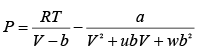...(2.21)

As part of a generalized approach to representing non-ideal gas behavior parameter Z, termed the compressibility factor, may be defined. This is discussed further in the following section. However, here we provide the expression for it:

Z = PV/RT                           ...(2.22)

Using eqn. 2.20, eqn. 2.19 may be rewritten as:

Z3 +αZ2 + βZ +γ= 0                            ...(2.23)

Where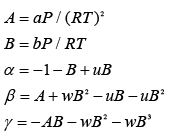Expressions for all the above parameters for each EOS are provided in Table 2.1 below.

Table 2.1 Values of u,w , α , β ,γ for various cubic EOSs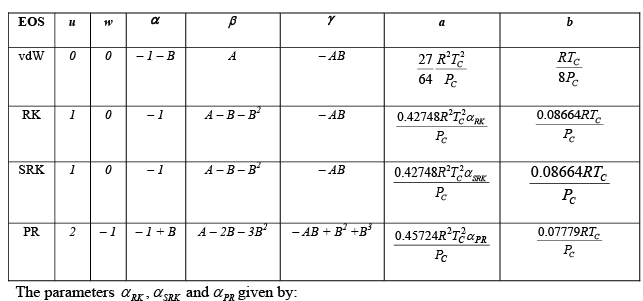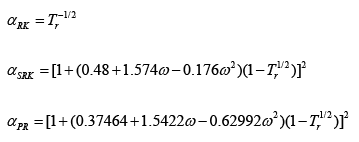As may be evident from table 2.1 all the parameters are determinable from the critical properties, ω and the temperature at which the molar volume or pressure needs to be calculated. Once these are computed the eqn. 2.21 may be solved to yield the value of Z, which in turn may be used to obtain the molar volume. Eqn. 2.21 may be solved using a suitable iterative algorithm or more readily by the general analytical solution for cubic algebraic equations, which is outlined below.

For solving eqn. 2.21, substitute, ( α / 3) which gives:

X3 + pX +q =0

where: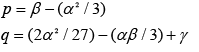Now, let (q2 / 4) ( p/ 27)

Then the roots of eqn. (2.21) are as follows:

Case 1:

If 0, then only one real root exists: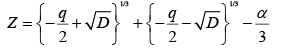Case 2 :

If 0, then there are three real roots, of which two are equal: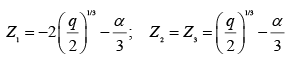Case 3:

If 0, then there are three unequal real roots: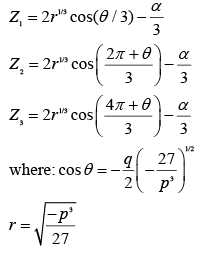The true advantage of use of cubic EOS derives from the fact that not only can it provide gas phase molar volumes, but also that of saturated liquid at a given temperature (note that this is not feasible by use of virial EOS). To understand this point we refer to fig. 2.6, which is a simplified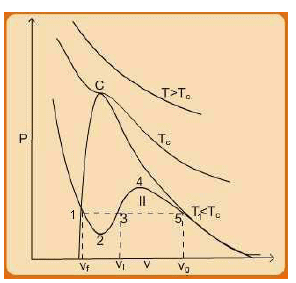Figure 2.6 P-V plots at constant temperatures (as obtained from a cubic equation of state)
version of fig. 2.1.

For given values of V and T one can calculate P (using any cubic EOS) as a function of V for various values of T. Figure 2.4 is a schematic P-V curves for three different temperatures. The variations shown by T = T C and T > T C have already been discussed in section 2.1. We draw your attention here to the plot at T < T C . In this case the pressure decreases rapidly in the subcooled liquid region with increasing V; after crossing the saturated-liquid line, it goes through a minimum (1-2-3), rises to a maximum (3-4-5), and then decreases, crossing the saturated-vapor line and continuing downward into the superheated-vapor region. However, experimentally obtained plots of this kind (at T < TC) do not show this manner of transition from saturated liquid to saturated vapor. As already discussed in section 2.1, in the two phase region within the dome, the curve connecting the saturated vapor and liquid states constitutes a tie-line. Along this horizontal segment the two phases coexist in varying proportions at the saturation vapor pressure. Clearly then the true behavior of the substance cannot be exactly reproduced by an analytical cubic EOS in the two phase region.

Nevertheless, the P-V behavior that obtains in the two-phase region using the cubic EOS is not entirely unrealistic. Indeed the two points of intersection of the analytical P-V curve (at a given temperature) with the boundary of the dome occurs (approximately) at the points where the experimental saturated vapour and liquid state volumes obtain (i.e., points 1 and 5). Cubic equations of state have three volume roots, of which two may often be complex conjugates.

Physically relevant values of V are always real, positive, and greater than constant b which appears in the cubic EOSs. For T > TC, at any pressure the solution for eqn. 2.21 yields one real root. This is also true at any pressure on the T = T C curve, except for P = P C ; at the latter condition three real roots obtain all equal to V C . For T < T C , in general there could be one or three real roots. If the pressure is different from the saturation vapour pressure (Psat, at the given temperature) the roots are not realistic. If, however, the pressure is taken to be Psat, three real roots are found. The highest amongst these correspond to the saturated vapour phase volume, while the lowest one approximates the saturated liquid phase volume; the third root, however, is not physically meaningful.

Principle of Corresponding States

The compressibility factor Z has already been defined in eqn. 2.20. A typical plot of Z as a function of T & P for methane is shown in fig.2.7. Experimentally measured values of Z for different fluids display similar dependence on reduced temperature and pressures, i.e., Pr andTr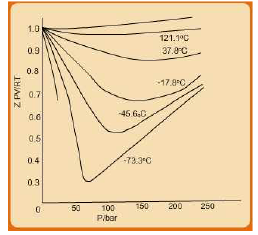Fig. 2.7 Variation of Compressibility-factor with pressure and temperature.

This observation has been generalized to formulate the two-parameter theorem of corresponding states which is stated as follows: “All fluids at the same reduced temperature and reduced pressure have approximately the same compressibility factor, and all deviate from ideal-gas behavior to about the same degree.” Fig. 2.8 presents select experimental data which support this observation.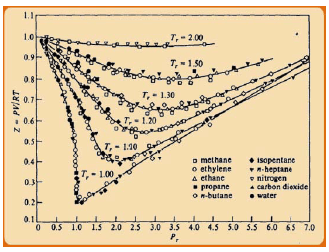Fig. 2.8 Experimental compressibility factors for different fluids as a function of the reduced temperature and pressure.

While this theorem applies fairly reliably to the simple fluids (argon, krypton, and xenon), for more complex fluids the deviations are significant. To address this gap Pitzer and coworkers introduced a third corresponding-states parameter, characteristic of molecular structure, more particularly the “degree of sphericity” of the molecule; the most widely used one is acentric factor ‘ω’ (already utilized in eqn. 2.13 and for computation for cubic EOS parameters in table 2.1).  The expression for ω was provided in eqn. 2.16. As is evident, it can be computed for any substance using critical properties and a single vapor-pressure measurement made at Tr = 0.7.

By definition ω is zero for the simple fluids argon, krypton, and xenon, which are generally regarded as spherical molecules. For other substances, the greater the deviation molecular sphericity, the larger is the departure of its corresponding ω from zero. For example for methane it is 0.012, while for butane it is 0.2, and so on. Experimentally determined values of Z for the three simply fluids coincide if measured at identical reduced temperature and pressures.  This observation forms the basis for extending the two-parameter theorem (stated above) to the three-parameter theorem of corresponding states: “The compressibility factor for all fluids with the same value of ω, when compared at the same reduced temperature and pressure are approximately the same, and hence the deviation from ideal-gas behavior is nearly the same.”

This theorem leads to vary convenient approach involving generalized correlations for computing not only the volumetric properties but for estimating a wide variety of other thermodynamic properties.

eneralized Compressibility factor Approach to EOS:  Pitzer Correlations For prediction of volumetric properties (using the compressibility factor Z or the second virial coefficient) the most commonly used correlations are those due to Pitzer and coworkers (op. cit.). According to this approach, compressibility factor is decomposed as follows: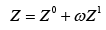......(2.24)

Where Z 0and Z 1 are both functions of Tr andPr only. When ω = 0, as for the simple fluids, the second term disappears. Thus the second term generally accounts for relatively small contribution to the overall Z due to the asphericity of a molecule. As noted earlier, the value of ω may be computed using the following equation

ω = −1.0− log( Prsat )Tr =0.7                  ......(2.16)

Fig. 2.9 shows a plot of reduced vapor pressures for select substances as a function of reduced temperature.  At a value of about ~ 0.7 for the reduced temperature (Tr) of the typical simple fluids (argon, krypton, xenon) the logarithm of reduced pressure is – 1. For other molecules the greater the departure from sphericity of the structure the lesser is the value of reduced pressure at Tr = 0.7. This is indicative of lower volatility of the substance, which suggests relatively stronger intermolecular interactions in the condensed phase. Stronger interactions result from higher polarity of molecules, which is in turn originates from the asymmetry of the molecular structure. Equation 2.16 indicates that the difference between the reduced pressures at the common reduced temperature ( 0.7) r T = is the measure for the acentric factor.

Based on the Pitzer-type correlations, Lee-Kesler has developed generalized correlations using a variant of the BWREOS (eqn. 2.17) for computing Z0 and Z1 as function of Tr andPr .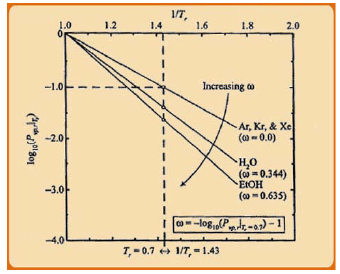Fig. 2.9 Plot of P r vs. T r for select substances of varying polarity (Source: J.W. Tester and M. Modell,
Thermodynamics and its Applications, 3rd ed., Prentice Hall, 1999).

The values of  Z0 and Z1 are available in the form of tables from where their values may be read off after due interpolation wherever necessary, or in the form of figures (see figs 2.10a and 2.10b).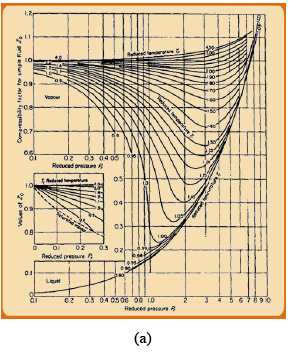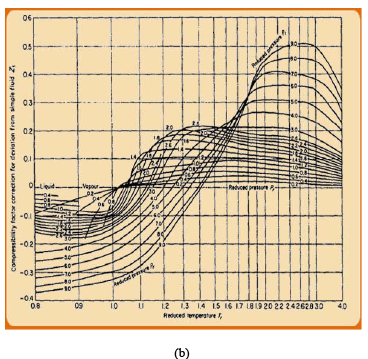Fig. 2.10a & 2.10b  Z0 and Z1 contributions to generalized corresponding states correlation developed by Pitzer and coworkers (1955)

Offer running on EduRev: Apply code STAYHOME200 to get INR 200 off on our premium plan EduRev Infinity!

## Thermodynamics

56 videos|92 docs|33 tests

,

,

,

,

,

,

,

,

,

,

,

,

,

,

,

,

,

,

,

,

,

;